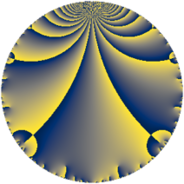# Properties

 Label 539.4.aLevel $539$ Weight $4$ Character orbit 539.a Rep. character $\chi_{539}(1,\cdot)$ Character field $\Q$ Dimension $102$ Newform subspaces $16$ Sturm bound $224$ Trace bound $5$

# Related objects

## Defining parameters

 Level: $$N$$ $$=$$ $$539 = 7^{2} \cdot 11$$ Weight: $$k$$ $$=$$ $$4$$ Character orbit: $$[\chi]$$ $$=$$ 539.a (trivial) Character field: $$\Q$$ Newform subspaces: $$16$$ Sturm bound: $$224$$ Trace bound: $$5$$ Distinguishing $$T_p$$: $$2$$, $$3$$

## Dimensions

The following table gives the dimensions of various subspaces of $$M_{4}(\Gamma_0(539))$$.

Total New Old
Modular forms 176 102 74
Cusp forms 160 102 58
Eisenstein series 16 0 16

The following table gives the dimensions of the cuspidal new subspaces with specified eigenvalues for the Atkin-Lehner operators and the Fricke involution.

$$7$$$$11$$FrickeDim.
$$+$$$$+$$$$+$$$$28$$
$$+$$$$-$$$$-$$$$20$$
$$-$$$$+$$$$-$$$$24$$
$$-$$$$-$$$$+$$$$30$$
Plus space$$+$$$$58$$
Minus space$$-$$$$44$$

## Trace form

 $$102 q + 2 q^{2} - 2 q^{3} + 392 q^{4} + 22 q^{5} - 10 q^{6} + 72 q^{8} + 888 q^{9} + O(q^{10})$$ $$102 q + 2 q^{2} - 2 q^{3} + 392 q^{4} + 22 q^{5} - 10 q^{6} + 72 q^{8} + 888 q^{9} + 62 q^{10} - 22 q^{11} + 132 q^{12} + 88 q^{13} + 150 q^{15} + 1648 q^{16} - 28 q^{17} - 16 q^{18} - 160 q^{19} - 60 q^{20} - 22 q^{22} - 130 q^{23} - 56 q^{24} + 2764 q^{25} + 588 q^{26} + 106 q^{27} + 232 q^{29} + 798 q^{30} + 546 q^{31} + 648 q^{32} + 154 q^{33} + 372 q^{34} + 3988 q^{36} - 1738 q^{37} + 604 q^{38} - 1248 q^{39} + 876 q^{40} - 352 q^{41} - 580 q^{43} - 528 q^{44} - 1264 q^{45} + 1314 q^{46} + 708 q^{47} + 884 q^{48} - 3504 q^{50} + 1100 q^{51} + 372 q^{52} - 1108 q^{53} - 3354 q^{54} + 418 q^{55} + 1040 q^{57} - 428 q^{58} + 1626 q^{59} + 3376 q^{60} - 1000 q^{61} - 330 q^{62} + 6076 q^{64} + 1224 q^{65} - 286 q^{66} + 58 q^{67} - 2452 q^{68} - 1846 q^{69} - 2286 q^{71} + 2396 q^{72} + 760 q^{73} + 3518 q^{74} - 1544 q^{75} + 1080 q^{76} + 5112 q^{78} + 2748 q^{79} - 996 q^{80} + 9094 q^{81} - 3816 q^{82} - 2460 q^{83} + 3436 q^{85} - 2408 q^{86} - 1968 q^{87} - 660 q^{88} + 4350 q^{89} + 10012 q^{90} - 1260 q^{92} + 1018 q^{93} - 572 q^{94} - 4912 q^{95} + 1524 q^{96} - 1366 q^{97} - 484 q^{99} + O(q^{100})$$

## Decomposition of $$S_{4}^{\mathrm{new}}(\Gamma_0(539))$$ into newform subspaces

Label Dim. $$A$$ Field CM Traces A-L signs $q$-expansion
$$a_2$$ $$a_3$$ $$a_5$$ $$a_7$$ 7 11
539.4.a.a $$1$$ $$31.802$$ $$\Q$$ None $$3$$ $$-4$$ $$-12$$ $$0$$ $$-$$ $$-$$ $$q+3q^{2}-4q^{3}+q^{4}-12q^{5}-12q^{6}+\cdots$$
539.4.a.b $$1$$ $$31.802$$ $$\Q$$ None $$3$$ $$-3$$ $$-2$$ $$0$$ $$-$$ $$-$$ $$q+3q^{2}-3q^{3}+q^{4}-2q^{5}-9q^{6}+\cdots$$
539.4.a.c $$1$$ $$31.802$$ $$\Q$$ None $$3$$ $$3$$ $$2$$ $$0$$ $$+$$ $$-$$ $$q+3q^{2}+3q^{3}+q^{4}+2q^{5}+9q^{6}+\cdots$$
539.4.a.d $$2$$ $$31.802$$ $$\Q(\sqrt{2})$$ None $$-2$$ $$4$$ $$4$$ $$0$$ $$-$$ $$+$$ $$q+(-1+\beta )q^{2}+2q^{3}+(1-2\beta )q^{4}+\cdots$$
539.4.a.e $$2$$ $$31.802$$ $$\Q(\sqrt{3})$$ None $$2$$ $$2$$ $$-2$$ $$0$$ $$-$$ $$+$$ $$q+(1+\beta )q^{2}+(1+4\beta )q^{3}+(-4+2\beta )q^{4}+\cdots$$
539.4.a.f $$4$$ $$31.802$$ 4.4.509800.1 None $$-4$$ $$12$$ $$18$$ $$0$$ $$-$$ $$-$$ $$q+(-1+\beta _{2})q^{2}+(3-\beta _{3})q^{3}+(6-\beta _{1}+\cdots)q^{4}+\cdots$$
539.4.a.g $$4$$ $$31.802$$ 4.4.522072.1 None $$-2$$ $$-14$$ $$-10$$ $$0$$ $$-$$ $$+$$ $$q+\beta _{2}q^{2}+(-4-\beta _{1})q^{3}+(6-\beta _{2}-2\beta _{3})q^{4}+\cdots$$
539.4.a.h $$5$$ $$31.802$$ $$\mathbb{Q}[x]/(x^{5} - \cdots)$$ None $$1$$ $$-2$$ $$24$$ $$0$$ $$-$$ $$-$$ $$q+\beta _{1}q^{2}+\beta _{3}q^{3}+(9+\beta _{1}+\beta _{2})q^{4}+\cdots$$
539.4.a.i $$6$$ $$31.802$$ 6.6.1536100192.1 None $$0$$ $$0$$ $$0$$ $$0$$ $$-$$ $$+$$ $$q-\beta _{5}q^{2}+\beta _{1}q^{3}+(1-\beta _{4})q^{4}+(-\beta _{1}+\cdots)q^{5}+\cdots$$
539.4.a.j $$9$$ $$31.802$$ $$\mathbb{Q}[x]/(x^{9} - \cdots)$$ None $$-3$$ $$-15$$ $$-32$$ $$0$$ $$+$$ $$-$$ $$q-\beta _{1}q^{2}+(-2+\beta _{1}+\beta _{4})q^{3}+(3+\beta _{2}+\cdots)q^{4}+\cdots$$
539.4.a.k $$9$$ $$31.802$$ $$\mathbb{Q}[x]/(x^{9} - \cdots)$$ None $$-3$$ $$15$$ $$32$$ $$0$$ $$-$$ $$-$$ $$q-\beta _{1}q^{2}+(2-\beta _{1}-\beta _{4})q^{3}+(3+\beta _{2}+\cdots)q^{4}+\cdots$$
539.4.a.l $$10$$ $$31.802$$ $$\mathbb{Q}[x]/(x^{10} - \cdots)$$ None $$-4$$ $$0$$ $$0$$ $$0$$ $$+$$ $$-$$ $$q-\beta _{5}q^{2}+(-\beta _{3}+\beta _{6})q^{3}+(\beta _{1}+\beta _{5}+\cdots)q^{4}+\cdots$$
539.4.a.m $$10$$ $$31.802$$ $$\mathbb{Q}[x]/(x^{10} - \cdots)$$ None $$0$$ $$0$$ $$-10$$ $$0$$ $$-$$ $$+$$ $$q+\beta _{1}q^{2}-\beta _{4}q^{3}+(5+\beta _{2})q^{4}+(-1+\cdots)q^{5}+\cdots$$
539.4.a.n $$10$$ $$31.802$$ $$\mathbb{Q}[x]/(x^{10} - \cdots)$$ None $$0$$ $$0$$ $$10$$ $$0$$ $$+$$ $$+$$ $$q+\beta _{1}q^{2}+\beta _{4}q^{3}+(5+\beta _{2})q^{4}+(1+\beta _{5}+\cdots)q^{5}+\cdots$$
539.4.a.o $$10$$ $$31.802$$ $$\mathbb{Q}[x]/(x^{10} - \cdots)$$ None $$4$$ $$0$$ $$0$$ $$0$$ $$-$$ $$-$$ $$q-\beta _{4}q^{2}+\beta _{8}q^{3}+(4-\beta _{5})q^{4}+(-\beta _{1}+\cdots)q^{5}+\cdots$$
539.4.a.p $$18$$ $$31.802$$ $$\mathbb{Q}[x]/(x^{18} - \cdots)$$ None $$4$$ $$0$$ $$0$$ $$0$$ $$+$$ $$+$$ $$q-\beta _{1}q^{2}-\beta _{3}q^{3}+(5-\beta _{7})q^{4}+\beta _{8}q^{5}+\cdots$$

## Decomposition of $$S_{4}^{\mathrm{old}}(\Gamma_0(539))$$ into lower level spaces

$$S_{4}^{\mathrm{old}}(\Gamma_0(539)) \cong$$ $$S_{4}^{\mathrm{new}}(\Gamma_0(7))$$$$^{\oplus 4}$$$$\oplus$$$$S_{4}^{\mathrm{new}}(\Gamma_0(11))$$$$^{\oplus 3}$$$$\oplus$$$$S_{4}^{\mathrm{new}}(\Gamma_0(49))$$$$^{\oplus 2}$$$$\oplus$$$$S_{4}^{\mathrm{new}}(\Gamma_0(77))$$$$^{\oplus 2}$$